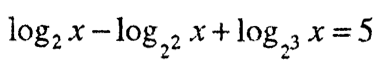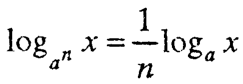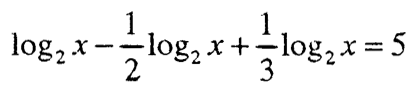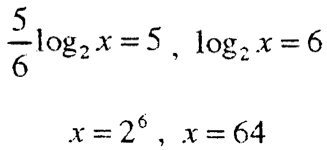# Logarithmic equations

An equation in which the unknown is in the logarithm or in the logarithmic basis of at least one logarithm is called a logarithmic equation.

There is no general method for solving a logarithmic equation.

Solving some types of logarithmic equations

1) Solve equations of the type logₐ x = b (a, b ∈ R).

If a> 0 and a ≠ 1, then for any real number b, the equation has a unique solution:2) Solving an equation of type (1)The solutions of this equation are obtained by solving the equation:

f (x) = g (x) (2)

Every solution of equation (1) is also a solution of equation (2), but the opposite is not true, so the solutions of equation (2) will be checked in equation (1).

Example 1. Let us solve the logarithmic equation

log₂ (x + 2) + log₂ (x + 14) = 6.

This equation is equivalent to the equation:

log₂ (x + 2) (х + 14) = log₂64

The solutions of the equation

(x + 2) (x + 14) = 64

are: х₁ = -18 and х₂ = 2.

Check. For x = -18, the solved equation passes into the equation

log₂ (-16) + log₂ (-4) = 6

which is untrue (logarithm cannot be a negative number). It follows that x = -18 is not a solution of the equation under consideration.

For the solution x = 2, the solved equation becomes an exact equation, ie.

log₂4 + log₂16 = 6, 2 + 4 = 6

So, x = 2 is a solution of the equation.

3) Solve an equation of type F (logₐ f (x)) = 0

In the equation F (logₐ f (x)) = 0

a> 0 and a ≠ 1, and f (x) is a given function of x. With the change logₐ f (x) = t it is reduced to the type: F (t) = 0

If the equation F (t) = 0 has solutions t₁, t₂, .… tk, then the solution of the given equation comes down to solving the set of logarithmic equations4) When solving some logarithmic equations, it is necessary to apply some of the known logarithmic identities.

Example 3. The equationcan be written like this:and applying identitythe equation is obtainedthat is###### – logarithmic equations –
Total Page Visits: 380 - Today Page Visits: 1